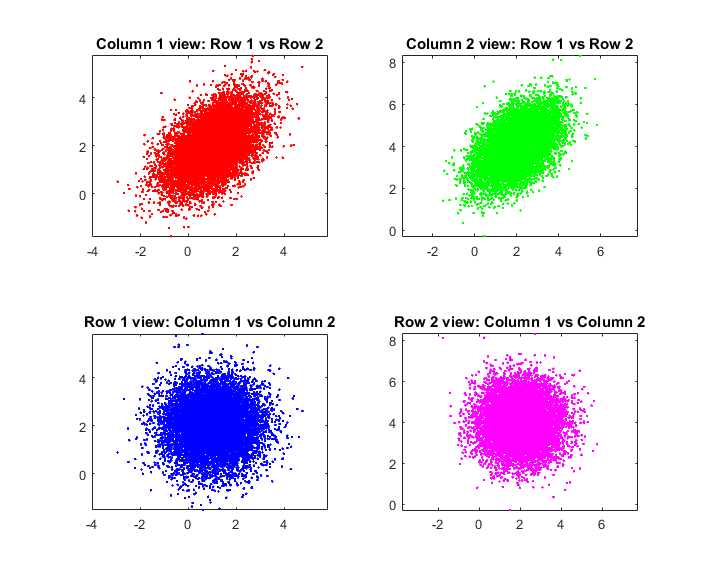PS：由于这部分直接跟矩阵代数相关，所以很多东西偶也只能陈述结论了哈，如果想要了解具体的只有自己好好钻研矩阵代数了哦！另外，确实这部分比较理论比较难，偶也只是尽量，尽量讲的通俗一点哈，不过看着好像还是很。。。

• 随机变量，随机向量，随机矩阵

A random variableis a measurable function from a set of possible outcomesto a measurable space. The technical axiomatic definition requiresto be a probability space (see Measure-theoretic definition). Usuallyis real-valued (i.e.).1. 均值矩阵：这个不用说，即对应的随机矩阵的各个元素的平均（期望）情况都要被均值矩阵中对应的元素所刻画。
2. 协方差矩阵：按照之前随机向量的讨论，我们需要协方差矩阵去刻画各个分量之间的关系（包括自身和自身的关系）。但是问题来了，对于一个向量，两两之间的关系用一个矩阵就可以刻画了，但是现在是一个矩阵，而就是说有d个列向量，此时，我们应该如何刻画这种关系呢？显然一个协方差矩阵是不够用了，所以我们现在需要两个矩阵，记为列协方差矩阵（column covariance matrix）和行协方差矩阵（row covariance matrix）。
对于随机矩阵，若我们按一列一列看，那么每一列就是一个随机向量，因为可以用对应的随机向量的协方差函数刻画。虽然这里一共有n行，但是我们可以说每一列虽然随机的，但是每一列事实上这个都是满足这个对应协方差的高斯分布的。所以之前所说的列协方差矩阵事实上也就是原先随机向量里的协方差矩阵。不过，单纯从列来看，我们是不是忽略了列与列之间的呢？对称来讲，我们还可以从行来看，一行一行看，其实这仍然可以看做一个随机向量，所以我们也可以用一个协方差矩阵去刻画它们之间的关系，这个就是在随机矩阵中的所谓的行协方差矩阵。1. 这个pdf你或许会见过好多个不同的表达形式，但本质都是一样的，主要原因是tr，也即是矩阵的迹的性质，至于你要问什么是矩阵的迹，这个只能wiki了一下矩阵的迹。首先矩阵的迹具有线性，即，另外就是矩阵的迹具有循环置换不变性，即。因此，上述的pdf还可以表述为其中表示迹的指数。
2. 别看这个这个表达式非常复杂，但是自然观察就可以发现这个公式非常有特色，也比较便于记忆。首先是常数项：的指数上的就是随机矩阵的size，行乘以列，常系数是-1/2，之后是列协方差矩阵与行协方差函数，这里需要重点强调的是，这两个矩阵的size,注意，这个的列协方差矩阵是的， 而行协方差函数是的哦！而在表示式中，却是有一种对角相乘的感觉哦，对应的系数是d而对应的系数是n，当然这个一直都有的-1/2也不能忘。只是etr里面的部分就是把各自看做整体然后至于哪个在前哪个在后其实关系并不大，看你自己的记忆习惯啦，当然为什么一个没有转置一个有转置，你想想这个矩阵乘法的要求就明白啦（size要匹配嘛！）

• 转置性

• 向量化% matrix Gaussian distribution
clc
clear
close all

seed=128; % set a random seed
rng(seed);
N = 10000; % the number of points

mu1 = [1;2];
sigma1 = [1 0;0 1];
mu2 = [2;4];
sigma2 = [1 0.5;0.5 1];

Mu = [mu1;mu2]; % produce a mean matrix using reshaping directly
Sigma = kron(sigma1,sigma2); % produce a new covariance matrix using kronecker product

sample = mvnrnd(Mu,Sigma,N); % generate a multivariate Gaussian distribition sample using
% the previous mean matrix and covariance matrix
sample = reshape(sample',2,2,N); % convert vector to matrix

% plot the matrix from columan and row veiw
subplot(2,2,1)
col1_view_row1 = reshape(sample(1,1,:),N,1);
col1_view_row2 = reshape(sample(2,1,:),N,1);
plot(col1_view_row1,col1_view_row2,'r.')
axis equal
title('Column 1 view: Row 1 vs Row 2')

subplot(2,2,2)
col2_view_row1 = reshape(sample(1,2,:),N,1);
col2_view_row2 = reshape(sample(2,2,:),N,1);
plot(col2_view_row1,col2_view_row2,'g.')
axis equal
title('Column 2 view: Row 1 vs Row 2')

subplot(2,2,3)
row1_view_col1 = reshape(sample(1,1,:),N,1);
row1_view_col2 = reshape(sample(1,2,:),N,1);
plot(row1_view_col1,row1_view_col2,'b.')
title('Row 1 view: Column 1 vs Column 2')
axis equal

subplot(2,2,4)
row2_view_col1 = reshape(sample(2,1,:),N,1);
row2_view_col2 = reshape(sample(2,2,:),N,1);
plot(row2_view_col1,row2_view_col2,'m.')
axis equal
title('Row 2 view: Column 1 vs Column 2')


• 其他各种线性性质

• 边际分布和条件分布

## 参考文献

1. Gupta A K, Nagar D K. Matrix variate distributions[M]. CRC Press, 1999.
2. Chen Z, Wang B, Gorban A N. Multivariate Gaussian and Student $-t$ Process Regression for Multi-output Prediction[J]. arXiv preprint arXiv:1703.04455, 2017.arxiv.org/pdf/1703.0445